Get inspired by the success stories of our students in IIT JAM MS, ISI  MStat, CMI MSc DS.  Learn More

# ISI MStat PSB 2009 Problem 8 | How big is the Mean?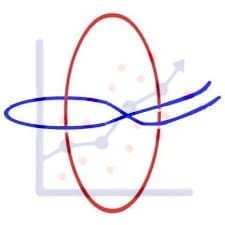This is a very simple and regular sample problem from ISI MStat PSB 2009 Problem 8. It It is based on testing the nature of the mean of Exponential distribution. Give it a Try it !

## Problem- ISI MStat PSB 2009 Problem 8

Let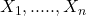be i.i.d. observation from the density,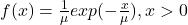where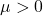is an unknown parameter.

Consider the problem of testing the hypothesis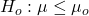against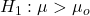.

(a) Show that the test with critical region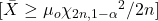, where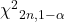is the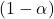th quantile of the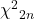distribution, has size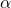.

(b) Give an expression of the power in terms of the c.d.f. of thedistribution.

### Prerequisites

Likelihood Ratio Test

Exponential Distribution

Chi-squared Distribution

## Solution :

This problem is quite regular and simple, from the given form of the hypotheses , it is almost clear that using Neyman-Pearson can land you in trouble. So, lets go for something more general , that is Likelihood Ratio Testing.

Hence, the Likelihood function of the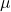for the given sample is ,, also observe that sample meanis the MLE of.

So, the Likelihood Ratio statistic is,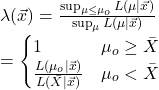So, our test function is ,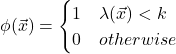.

We, rejectat size, when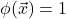, for some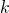,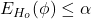,

Hence,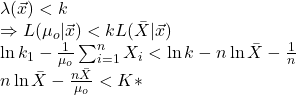.

for some constant,.

Let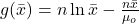, and observe that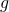is,

decreasing function of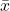for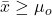,

Hence, there exists a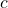such that,we have. See the figure.

So, the critical region of the test is of form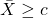, for somesuch that,, for some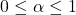, whereis the size of the test.

Now, our task is to find, and for that observe, if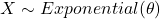, then,

Hence, in this problem, since the's follows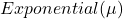, hence,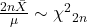, we have,,

which gives,

Hence, the rejection region is indeed,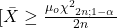.

Hence Proved !

(b) Now, we know that the power of the test is,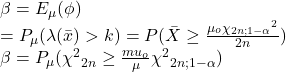.

Hence, the power of the test is of form of a cdf of chi-squared distribution.

## Food For Thought

Can you use any other testing procedure to conduct this test ?

## Subscribe to Cheenta at Youtube

This is a very simple and regular sample problem from ISI MStat PSB 2009 Problem 8. It It is based on testing the nature of the mean of Exponential distribution. Give it a Try it !

## Problem- ISI MStat PSB 2009 Problem 8

Letbe i.i.d. observation from the density,whereis an unknown parameter.

Consider the problem of testing the hypothesisagainst.

(a) Show that the test with critical region, whereis theth quantile of thedistribution, has size.

(b) Give an expression of the power in terms of the c.d.f. of thedistribution.

### Prerequisites

Likelihood Ratio Test

Exponential Distribution

Chi-squared Distribution

## Solution :

This problem is quite regular and simple, from the given form of the hypotheses , it is almost clear that using Neyman-Pearson can land you in trouble. So, lets go for something more general , that is Likelihood Ratio Testing.

Hence, the Likelihood function of thefor the given sample is ,, also observe that sample meanis the MLE of.

So, the Likelihood Ratio statistic is,So, our test function is ,.

We, rejectat size, when, for some,,

Hence,.

for some constant,.

Let, and observe thatis,

decreasing function offor,

Hence, there exists asuch that,we have. See the figure.

So, the critical region of the test is of form, for somesuch that,, for some, whereis the size of the test.

Now, our task is to find, and for that observe, if, then,

Hence, in this problem, since the's follows, hence,, we have,,

which gives,

Hence, the rejection region is indeed,.

Hence Proved !

(b) Now, we know that the power of the test is,.

Hence, the power of the test is of form of a cdf of chi-squared distribution.

## Food For Thought

Can you use any other testing procedure to conduct this test ?

## Subscribe to Cheenta at Youtube

This site uses Akismet to reduce spam. Learn how your comment data is processed.

### Knowledge Partner